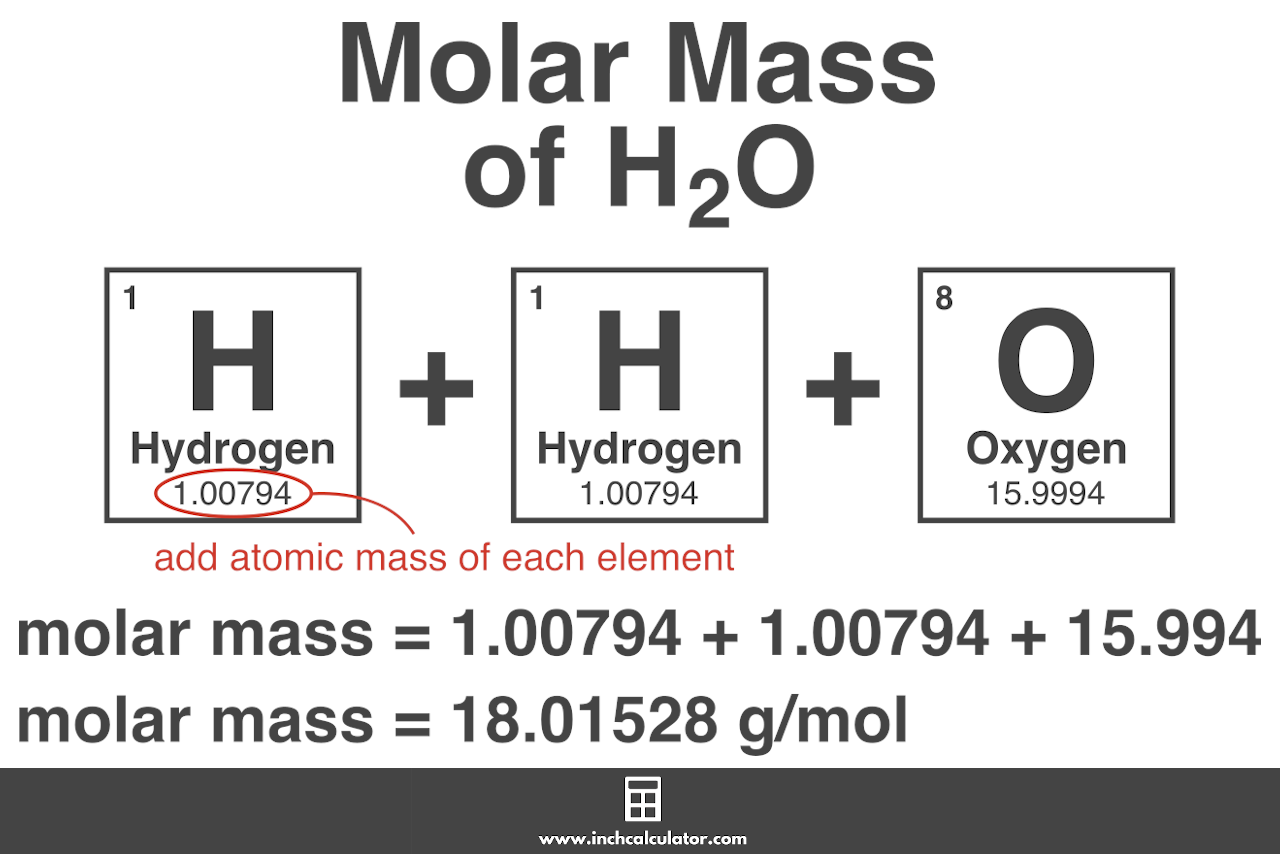# Molar Mass Practice Problems Study Guide

INTRODUCTION

Have you ever wondered how many air molecules there are in the soccer ball you’re playing with or the food you’re eating? Atoms or molecules are the smallest units in terms of mass and size, as we all understand. The molar mass is the mass of one sampling mole. The molar mass of a compound is computed by summing the atomic masses of all the atoms in the molecule. So, how do you estimate the molar mass mathematically?

### WHAT IS MOLAR MASS?

The molar mass of any substance is defined as the smallest mass unit of that compound with one-twelfth of the mass of one carbon that would be 12 atoms. In any substance, the number of entities present can always be defined as a mole.Source

### HOW TO CALCULATE THE MOLAR MASS OF A COMPOUND?

• Using the periodic table, find the atomic masses. For each element, you must figure out the atomic mass using the periodic table. For any element, the number that appears on the top left represents atomic mass.
• Count the number of atoms present in each element (The subscript of an element in its chemical formula).
• Now find the molar mass. Now that you know the atomic mass and how many atoms of the compound are involved, you can find the molar mass as shown below.Source

Calculate the molar mass of Na₂CO₃:

Na: 2 x 23.0 = 46

C: 1 x 12.0 = 12

O: 3 x 16 = 48

To get the final answer, add up the total values, i.e., 46 12 48 = 106Hence, the molar mass of Na₂CO₃ is 106 g/mole.

Let’s take another example to find the mass of sulfur in an 8.5 mol sample of sulfur:

mass of S = moles of S × molar mass of S

mass of S = 8.5 mol S × 32.07 g/mol S

mass of S = 272.595 g

Here are some questions for you to practise:

1. Find the molar mass of CaCOH
2. Find the molar mass of Cr₄(P₂O7)₃
3. Calculate the molar mass of KAl(SO₄)₂·12 H₂O
4. Find the number of moles in a 39.25-gram sample of CdBr₂.

## CONCLUSION:

• Molar mass can be defined as the smallest unit of mass of any compound and is the twelfth of one carbon mass – 12 atoms.
• The standard unit is g mol⁻¹ and the SI one as kg mol⁻¹.

### FAQs

1. How do you find the molar mass?

• Calculate the number of atoms of every element in the compound using the chemical formula.
• Multiply every element’s atomic weight by the number of atoms included in the compound.
• Add everything up and convert to grams/mole.

2. What is molar mass in simple words?

The mass of a specimen of a chemical compound divided by the amount of material in that specimen, expressed in moles, is the molar mass of that compound.

3. What is molar mass, and what is its unit?

The mass in grams of one mole of a material is known as molar mass. Molar mass is measured in grams per mole.

We hope you enjoyed studying this lesson and learned something cool about Molar Mass Practice Problems! Join our Discord community to get any questions you may have answered and to engage with other students just like you! Don’t forget to download our App to experience our fun VR classrooms – we promise, it makes studying much more fun! 😎

## SOURCES

1. Molar Mass Formula: https://byjus.com/molar-mass-formula/ Accessed 14 march 2022
2. Molar Mass Q&A: https://study.com/learn/molar-mass-questions-and-answers.html Accessed 14 march 2022
]]>